# Dividing by five and ten

Number 5040 divide by the number 5 and by number 10:

a = 5040: 5
b = 5040: 10

a =  1008
b =  504

### Step-by-step explanation:

$a=5040\mathrm{/}5=1008$
$b=5040\mathrm{/}10=504$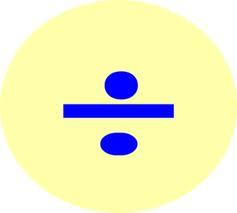Did you find an error or inaccuracy? Feel free to write us. Thank you!## Related math problems and questions:

• DivideDivide substance 110 cm long to two parts so that it first part is 10 cm longer than the second part and one part will be 10 times longer than the second portion. How long the parts will be?
• A koala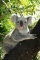A koala lives to be 14 years old in the wild. How much longer will a 2-year-old koala probably live?
• Unknown number 2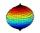I think the number. When he reduces it four times, I'll get 11. What number am I thinking?
• Mysterious number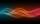The magician thinks the number: "The mysterious number is first divided by minus five, dividing the result by three, multiplying the number by ten, and dividing the resulting number by minus four. This gives result 5. Can you reveal the mysterious number?
• I thinkI think the number. If I add the number 983 to it and divide it by 6, I get the number 1428. What number do I think?
• Subtraction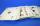How many times you can subtract the number 4 from the number 64?
• Written numberPlace+values x ten thousands =30 thousands
• DivideHow many different ways can three people divide 7 pears and 5 apples?
• Evaluate 11Multiply the quotient of 6 and 2 by 3, then add 1. Add 1 and 6, then divide by 2 and multiply by 3. Divide 6 by the product of 2 and 3, then add 1.Add marks (+, -, *, /, brackets) to fullfill equations 1 3 6 5 = 10 This is for the 4th grade of the primary school - with no negative numbers yet
• DivisionWhich number in division 16 give 12 and the rest 3?
• Bus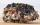On the 4-th stop take on 56 and take off 38 passengers. How many were added (write as positive number) or shrunk (write as negative number) the count of passengers?
• Big number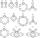The hat is the remainder when dividing number 10 to 47 - 111 by number 9?
• Unknown number xyzFind the number that its triple is 24. Solve by equation.
• Parenthesis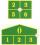Fill in the examples correctly brackets: A) 7.9+12:3-2 =23 B) 7.9+12:3-2=75
• Divide 13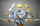Divide. Simplify your answer and write as an improper fraction or whole number. 14÷8/3
• Cakes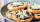On the table was 56 cakes. When Peter went home, leaving them eight times less. How many cakes left?